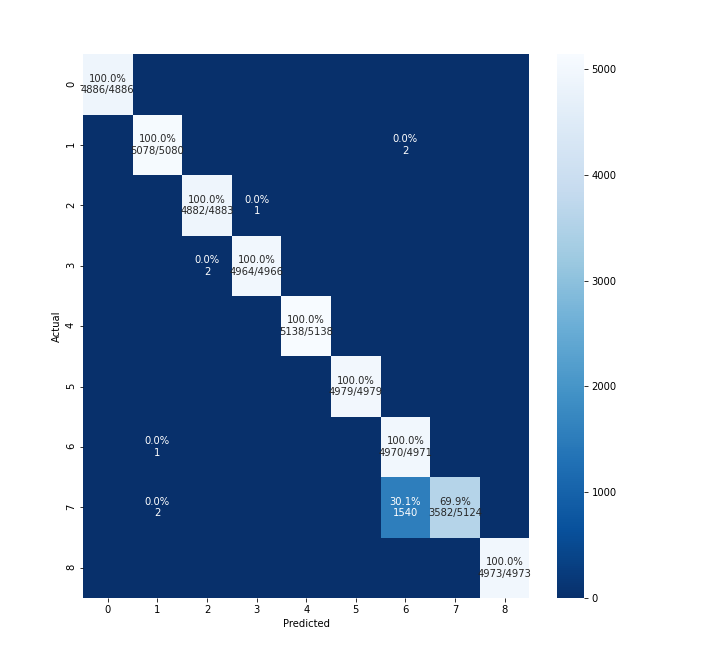Instantly share code, notes, and snippets.

# hitvoice/plot_confusion_matrix.png

Last active June 15, 2023 12:39
Star You must be signed in to star a gist
Generate matrix plot for confusion matrix with pretty annotations.This file contains bidirectional Unicode text that may be interpreted or compiled differently than what appears below. To review, open the file in an editor that reveals hidden Unicode characters. Learn more about bidirectional Unicode characters
 import numpy as np import pandas as pd import matplotlib.pyplot as plt import seaborn as sns from sklearn.metrics import confusion_matrix def cm_analysis(y_true, y_pred, filename, labels, ymap=None, figsize=(10,10)): """ Generate matrix plot of confusion matrix with pretty annotations. The plot image is saved to disk. args: y_true: true label of the data, with shape (nsamples,) y_pred: prediction of the data, with shape (nsamples,) filename: filename of figure file to save labels: string array, name the order of class labels in the confusion matrix. use `clf.classes_` if using scikit-learn models. with shape (nclass,). ymap: dict: any -> string, length == nclass. if not None, map the labels & ys to more understandable strings. Caution: original y_true, y_pred and labels must align. figsize: the size of the figure plotted. """ if ymap is not None: y_pred = [ymap[yi] for yi in y_pred] y_true = [ymap[yi] for yi in y_true] labels = [ymap[yi] for yi in labels] cm = confusion_matrix(y_true, y_pred, labels=labels) cm_sum = np.sum(cm, axis=1, keepdims=True) cm_perc = cm / cm_sum.astype(float) * 100 annot = np.empty_like(cm).astype(str) nrows, ncols = cm.shape for i in range(nrows): for j in range(ncols): c = cm[i, j] p = cm_perc[i, j] if i == j: s = cm_sum[i] annot[i, j] = '%.1f%%\n%d/%d' % (p, c, s) elif c == 0: annot[i, j] = '' else: annot[i, j] = '%.1f%%\n%d' % (p, c) cm = pd.DataFrame(cm, index=labels, columns=labels) cm.index.name = 'Actual' cm.columns.name = 'Predicted' fig, ax = plt.subplots(figsize=figsize) sns.heatmap(cm, annot=annot, fmt='', ax=ax) plt.savefig(filename)

### kalkite commented Oct 11, 2021 • edited

@hitvoice, it's really appreciated work. how to changet the `clf.classes_` to other, for instance my classess are from 0 to 8, but i want to change those labels to string.### hitvoice commented Oct 17, 2021

@rajeshkalakoti pass the value of `ymap` (dict[Any,str]) to `cm_analysis`. You can configure the class names in `ymap`.

### kalkite commented Oct 24, 2021

for instance, labels are like "class-1, class-2,class-3, class-4,class-5, class-6,class-7, class-8,classs-9", how to configure it?